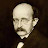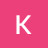Problem 14, January 2017 Algebra I (Common Core) Regents Exam – JuanTutors.comProblem 14, January 2017 Algebra I (Common Core) Regents Exam – JuanTutors.com

14 A computer application generates a sequence of musical notes using the function f(n) = 6(16)^n, where n is the number of the note in the sequence and f(n) is the note frequency in hertz. Which function will generate the same note sequence as f(n)?
(1) g(n) = 12(2)^(4n) (3) p(n) = 12(4)^(2n)
(2) h(n) = 6(2)^(4n) (4) k(n) = 6(8)^(2n)

algebra tutors,Math,Regents,Algebra I,Algebra 1,Tutoring,JuanTutors,JuanTutors.com,Juan,Juan Tutors,Exponential Functions,Laws of Exponents

algebratutors.org

1.Alexander Garfin says:
2.Kim Long says: